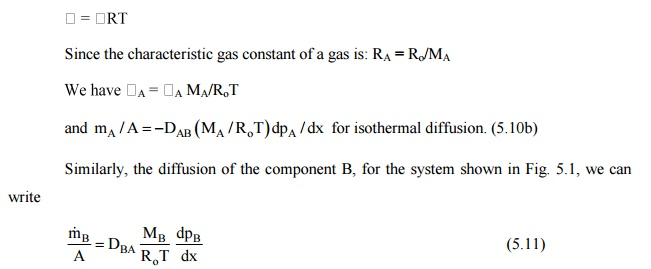Home | | Heat and Mass Transfer | Heat and Mass Transfer Analogy

# Heat and Mass Transfer Analogy

Convective Mass Transfer Correlations, Heat And Mass Transfer Analogy

HEAT AND MASS TRANSFER ANALOGY:

Fick's law (Eq. 5.8) can also be expressed in terms of mass flux per unit area or mass concentration or in terms of molal concentrations and fluxes. For gases, the law may be expressed in terms of partial pressures by making use of the perfect gas equation of state:

Since the characteristic gas constant of a gas is: RA = Ro/MAWhen we have equimolal counter diffusion, shown in Fig. 12.3 (a, b), the steady state molal diffusion rates of the species A and B, represented by NA and NB will be given byThe total pressure of the system remains constant at steady state,

or, p = pA + pB ;  and dpA/dx + dpB/dx = 0

as  dpA/dx = –dpB/dx

Since each molecule of A is replaced by a molecule of B, the molal diffusion rates must be equal. Thus: NA = –NB, andOr DAB = DBA = D

This fact is known as the equivalence of diffusion coefficients or diffusivities in binary mixtures, and is a property of the binary mixture.

By integrating Eq. (12.10), we can obtain the mass flux of the species A as;corresponding to the nomenclature used in Fig. 5.3 (a, b). Table 5.2 gives the values of the binary diffusion coefficients.Fig. 5.3(b) Equlmolal counter-diffusion (partial pressure profile)

(iv) It does not tell about diffusion due to temperature or pressure gradient or due to external forces.

CONVECTIVE MASS TRANSFER CORRELATIONS :

Let us consider a tank containing water which is exposed to air in the tank as shown in Fig. 5.4. We assume that:

(i) the system is isothermal,

(ii) the total pressure remains constant,

(iii) the system is in steady state. Since there has to be a little movement of air over the top of the tank to remove the water vapour that diffuses to that point, the air movement does not create any turbulence to alter the concentration profile in the tank, and

air and water vapour both behave like ideal gases.From Eq. (5.10), the downward diffusion of air can be written asand this has to be balanced by the bulk mass transfer upward. Therefore,Study Material, Lecturing Notes, Assignment, Reference, Wiki description explanation, brief detail
Mechanical : Heat and Mass Transfer : Mass Transfer : Heat and Mass Transfer Analogy |